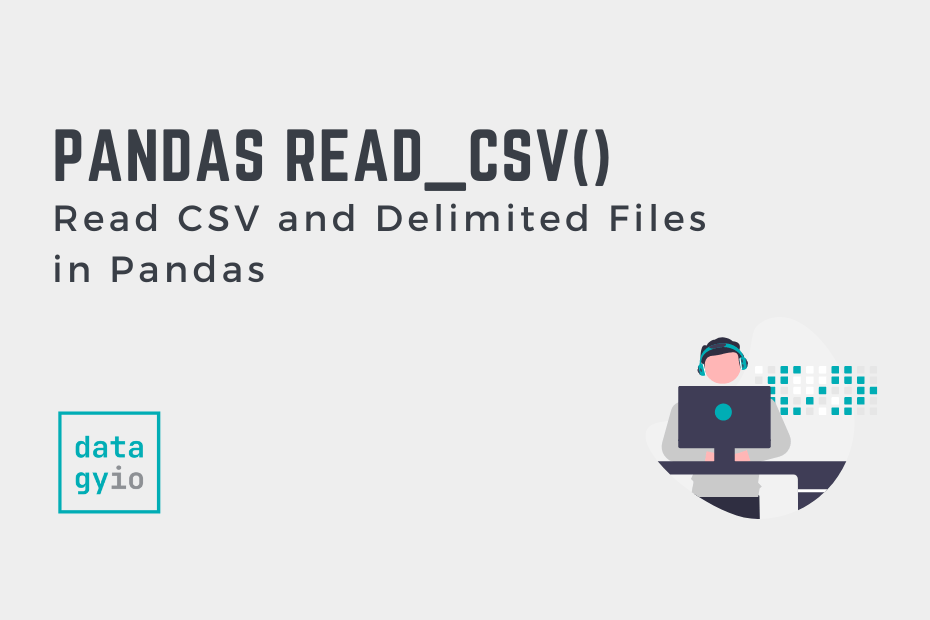# BlogIn this tutorial, you’ll learn how to use the Pandas read_csv() function to read CSV (or other delimited files) into DataFrames. CSV files are a ubiquitous file format that you’ll encounter regardless of the sector you work in. Being able to read… Read More »Pandas read_csv() – Read CSV and Delimited Files in Pandas## Using Python Generators and yield: A Complete Guide

In this tutorial, you’ll learn how to use generators in Python, including how to interpret the yield expression and how to use generator expressions. You’ll learn what the benefits of Python generators are and why they’re often referred to as… Read More »Using Python Generators and yield: A Complete Guide## Seaborn Regression Plots with regplot and lmplot

In this tutorial, you’ll learn how to use Seaborn to plot regression plots using the sns.regplot() and sns.lmplot() functions. It may seem confusing that Seaborn would offer two functions to plot regressive relationships. Don’t worry – this guide will simplify… Read More »Seaborn Regression Plots with regplot and lmplot## Seaborn residplot – Plotting Residuals of Linear Regression

In this tutorial, you’ll learn how to create a residual plot using Seaborn by using the sns.residplot() function. Residual plots let you evaluate the residuals of a regression fit by easily understanding their differences. By the end of this tutorial,… Read More »Seaborn residplot – Plotting Residuals of Linear Regression## Seaborn jointplot() – Creating Joint Plots in Seaborn

In this tutorial, you’ll learn how to use the Seaborn jointplot() function to create informative joint plots. Joint plots allow you to create helpful visuals that plot both a bivariate distribution (such as a scatter plot), as well as the… Read More »Seaborn jointplot() – Creating Joint Plots in Seaborn## Seaborn displot – Distribution Plots in Python

In this tutorial, you’ll learn how to create Seaborn distribution plots using the sns.displot() function. Distribution plots show how a variable (or multiple variables) is distributed. Seaborn provides many different distribution data visualization functions that include creating histograms or kernel… Read More »Seaborn displot – Distribution Plots in Python## Seaborn ecdfplot – Empirical Cumulative Distribution Functions

In this guide, you’ll learn how to use the Seaborn ecdfplot() function to create empirical cumulative distribution functions (ECDF) to visualize the distribution of a dataset. ECDF plots are valuable tools to visualize how datasets are distributed, allowing you to… Read More »Seaborn ecdfplot – Empirical Cumulative Distribution Functions## Seaborn rugplot – Plotting Marginal Distributions

In this guide, you’ll learn how to use the Seaborn rugfplot() function to plot distributions in the margins to visualize the distribution of a dataset. Rug plots are valuable tools to visualize how datasets are distributed, allowing you to gain… Read More »Seaborn rugplot – Plotting Marginal Distributions## Seaborn kdeplot – Creating Kernel Density Estimate Plots

In this guide, you’ll learn how to use the Seaborn histplot() function to create histograms to visualize the distribution of a dataset. Histograms are valuable tools to visualize how datasets are distributed, allowing you to gain strong insight into your… Read More »Seaborn kdeplot – Creating Kernel Density Estimate Plots## Seaborn histplot – Creating Histograms in Seaborn

In this guide, you’ll learn how to use the Seaborn histplot() function to create histograms to visualize the distribution of a dataset. Histograms are valuable tools to visualize how datasets are distributed, allowing you to gain strong insight into your… Read More »Seaborn histplot – Creating Histograms in Seaborn## Seaborn catplot – Categorical Data Visualizations in Python

In this tutorial, you’ll learn how to create Seaborn relational plots using the sns.catplot() function. Categorical plots show the relationship between a numerical and one or more categorical variables. Seaborn provides many different categorical data visualization functions that cover an… Read More »Seaborn catplot – Categorical Data Visualizations in Python## Seaborn Pointplot: Central Tendency for Categorical Data

In this tutorial, you’ll learn how to use the Seaborn pointplot function to create point plots. Point plots provide similar functions as bar plots, but provide more information, such as error bars around a measure of central tendency. That may… Read More »Seaborn Pointplot: Central Tendency for Categorical Data